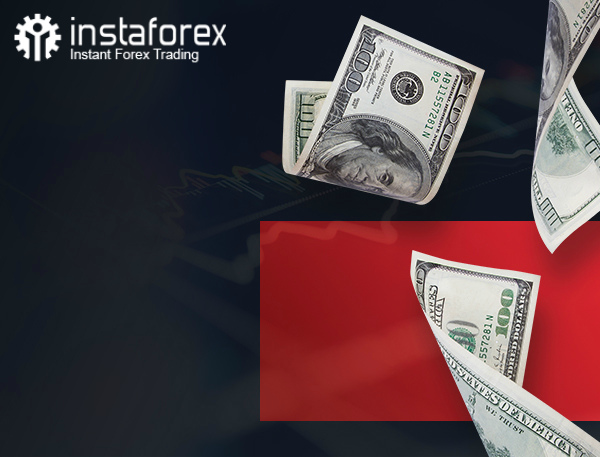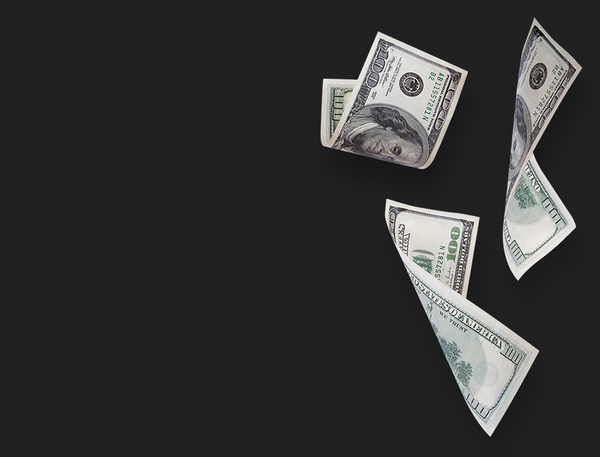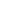no risks and investments
With new Start-Up Bonus of \$1000Get bonus
55%
from InstaForex
on every depositEarn up to
\$50000
for inviting friends to get StartUp Bonus from InstaForex
No investments required!
\$1000
No deposit bonus
Registration in 15 seconds without verification
Automatic bonus charging
Get bonus

# Lesson 28: the Fibonacci sequence is a magical instrument for traders

Published 16.09.2016 at 04.15 PM.

The Fibonacci sequence is one of the most popular trading instruments with forex traders. The developers of the MetaTrader platform had a good reason to put this instrument onto the toolbar along with such widely-used tools of technical analysis as lines and equidistant channel. Why is the Fibonacci sequence so popular in the forex market? It’s a common knowledge that traders are very superstitious, so they are disposed to employ the theory of Leonardo Fibonacci, the mathematician who introduced his golden section and the sequence of numbers: 0, 1, 1, 2, 3, 5, 8, 13, 21, 34, 55, 89, 144...

From the point of view of Fibonacci’s adherents, this law describes proportions which define harmony. Importantly, the harmony structure has a form of a spiral.

To prove this hypothesis, they provide examples from the nature: fingers length difference, a shape of a snail shell and some plants:

Aloe plurifoliateNautilus FossilSpiral GalaxyLearning about such a regularity, even a stubbornskeptic is likely to believe in God's purpose and the magic of the Fibonacci numbers. Let alone traders who are quite superstitious and sensitive to bad lucks such as a black cat.

A hen and an egg: which one came first?

In the endless number of models, there should be the one the motion of which corresponds to the certain formation rule. I suppose, that at some point those who were interested in promotion of the golden section marketed the models implying accurate application of the Fibonacci levels. The platform developers noticed this peculiarity and placed the Fibonacci instrument onto the toolbar. Interestingly, a price corresponds to these levels with ever increasing frequency. One would say, it’s a magic. I’d rather not. A price doesn’t correspond to the Fibonacci levels, but to clusters of orders which traders set at these levels. Thus, traders believe in the magic of the Fibonacci numbers and turn it into reality by their actions. Well, if this instrument helps to reveal trends, why not benefit from it? Sticking to certain rules, a trader can achieve good results with the use of the Fibonacci numbers. Let’s see how to apply this tool to define the correction depth and set targets for profit fixation.

1. Defining a rebound depth.

Here we use apercentage ratio which is calculated by the Fibonacci numbers division.

1 / 2 = 0.5

34 / 55 = 0.618

34 / 89= 0.382

34 / 144= 0.236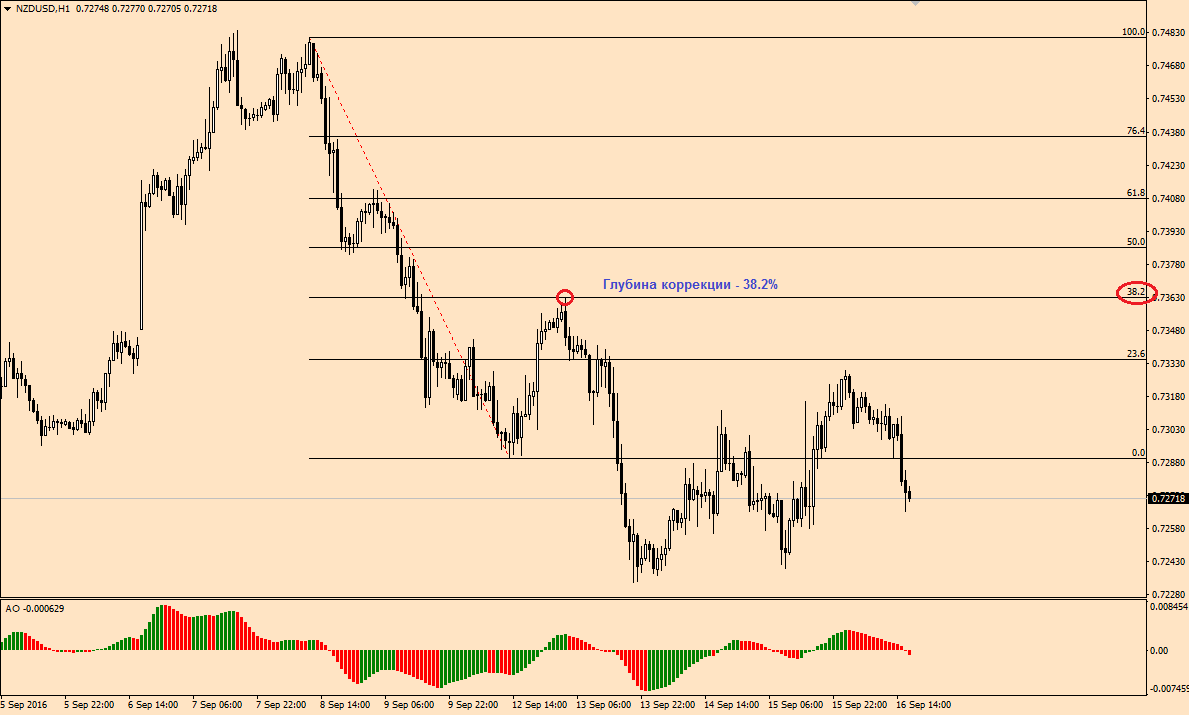2. Setting targets for profit fixation.

The ratio is calculated in a similar way to the correction levels.

2 / 1 = 2

55 / 34 = 1.62

89 / 34 = 2.62

144 / 34 = 4.23

I think, you’ve noticed that the 1.236 and 1.382 levels don’t exist, but traders use them actively, so a price corresponds to them.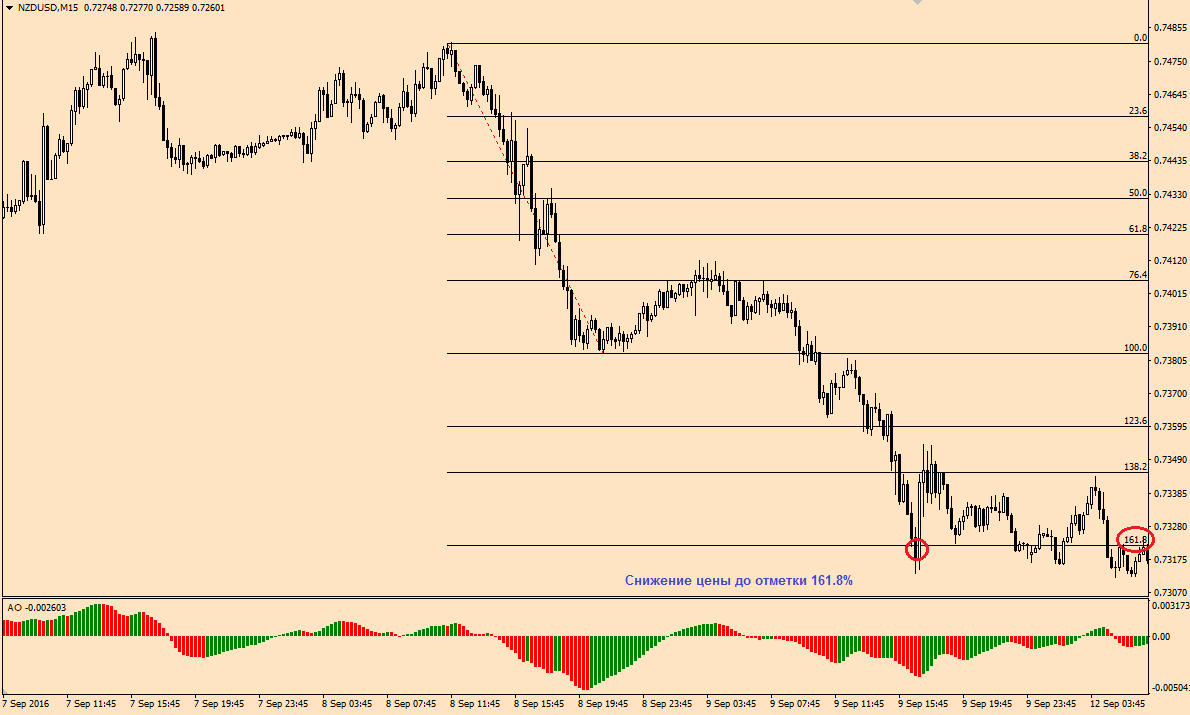In the next article we will have a closer look at how to apply the Fibonacci numbers in MetaTrader.